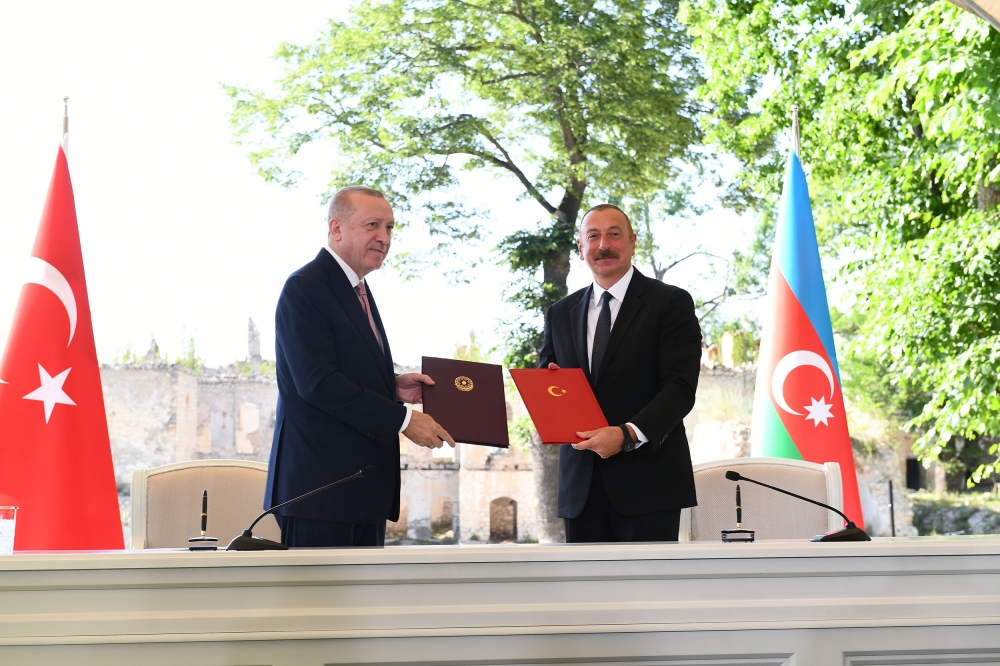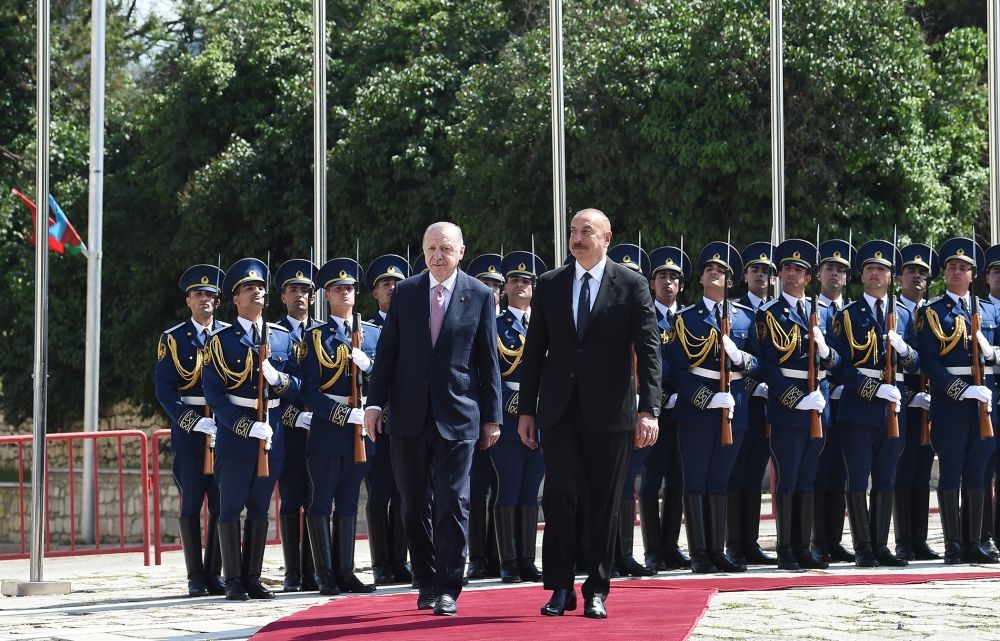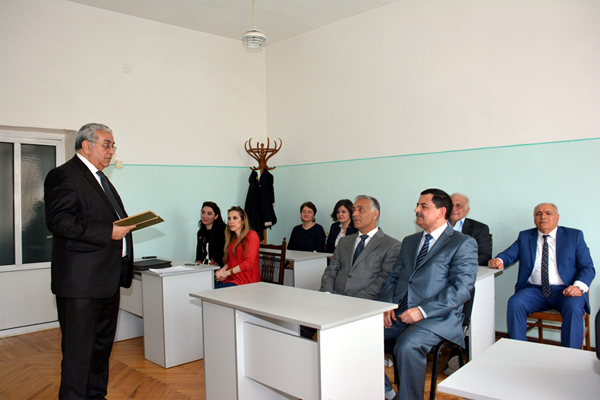Institute of Mathematics and Mechanics
Azerbaijan National Academy of Science

## Karabakh news### “Musical heritage and Karabakh horses on Jidir Duzu plain” composition organized by Heydar Aliyev Foundation was presented in Shusha### Azerbaijan, Turkey signed Shusha Declaration on allied relations## Department of “Differential equations”Head of the department: Aliyev Akbar Bayram oglu Doctor of Physical and Mathematical Sciences, Professor Tel: (050) 613-89-80 E-mail: alievakbar@gmail.com Number of employees: 10 The main scientific priorities of department: – Determination of the Cauchy problem for partial linear and nonlinear equations and systems, criteria of local and non-local solvability of a boundary value problem and mixed problem, study of smoothness of solutions and asymptotics. Determination of no global solvability criteria. – Determination of well posedness of the Cauchy problem and boundary value problem for operator coefficient equations. Determination evolution operator for higher order variable operator equation. – Study of spectral problems with a spectral parameter in the boudary condition for differential operator. – Study of Cauchy problem for Volterra chains. The obtained basic scientific results: In the department researchs are conducted in the following directions:-To establish   the criteria for local and global solvability of Cauchy problem, boundary value problem and mixed problem for linear and nonlinear equations and system of equations, investigation of asymptotics and smoothness of solutions.  To establish nonexistence criteria of global solution . -To establish correctness of Cauchy and boundary value problems for equations with operator coefficient. Determination of evaluation operator for higher order differential equations with variable operator coefficient. -Investigation of  spectral problems with eigenvalue dependent boundary condition. -The problems of optimal control for partial differential equations and differential equations with operator coefficient. The main results obtained: The following main results were obtained for the first problem. – Fujita type criteria for the existence of global solutions of the Cauchy problem for a system of semi-linear fractal dissipation hyperbolic equation and a semi- linear hyperbolic equations with quasielliptic part, were obtained. Conditions on the existence and absence of global solutions of the Cauchy problem for a system of semi-linear nonlinear dissipation hyperbolic equatond were determined; – Well-posedness of the Cauchy problem was determined for equations where a pary of coefficients are smooth, another part is nonsmooth; – Theorems on behaviour of solutions of nonlinear elliptic and parabolic equations in the vicinity special points of boundaries and in unbounded domains with noncompact boundary, were proved. When in bounded and in unbounded domains the weight function satiesfies the Mackenhoupt conditions, the degenerating coefficients satisfy certain growth conditions, the guality character of the solutions of Dirichlet problem stated for higher order nonlinear parabolic equations was studied and unigueness classes for them were found. – Quality properties of a solutions of certain class of elliptic and parabolic type equations were studied, sufficient conditions for almost periodicity and asymptotically of solutions of operator coefficient equations and partial hyperbolic equations were determined; Global solvability conditions of one-sided problemss for quasilinear hyperbolic equations were determined; – Radiation principles in higher order elliptic equations in cylindric domains were proved; resonans effect in justification of the limit amplitude principle was revealed, the Cauchy problem, the solutions of mixed problems were studied for Sobolev equations and for a system of well-posed by Petrovsky equations in bounded domains and in unbounded cylindric domains, and the asympthotics of solutions at layest values of time was reserached. The following results were obtained on the second problem. – The well-posedness of the Cauchy problem and the initial boundary value problem was studiedd for higher order variable operator coefficient evolution equations; – Fredholm property of solvability of a boundary value problem was studied for a second order differential-operator equation with an unbounded operator in the boundary condition. The following results were obtained on the third problem. – The trace of a boundary value problem was calculated for operator coefficient Sturm-Liouville equation and an unbounded operator differential operators with a spectral parameter in boundary conditions; – Solvability, Fredholm property and spectral pecularities of boundary value problems were studied for a second order differential-operator equation with a spectral parameter in the boundary condition. The following results were obtained on the fourth problem. -The Cauchy problem was studied for Volterra chains with asymptotic periodic initial conditions and was solved by the method of the inverse spectral problem. Research works published over above stated problems: -more than 150 research works regarding the 1 st problem are published in the scientific journals more than 70 of which  with high impact factor; – more than 100 research works regarding the 2 -nd  problem are published in the scientific journals more than 35 of which  with high impact factor; – more than 100 research works regarding the third problem are published in the scientific journals more than 35 of which  with high impact factor . – regarding the forth  problem several research works are published. Participation in scientific conferences A.A.Aliyev , M.Bayramoglu and B. Isgenderov were members of organazational  and program comitees of several international  conferences. All of our employees were participants of  many international conferences. Grant projects: Employees of department were participants of the following grant projects.– DOPROG  program Turkey, Afyon University(Ə.B.Əliyev-1997); – Participant of international scientific project “Physical and Geographical Model of the transgression diffusion of       pollutants in different Hydrometeorological Conditions on the Caspian Sea” CRDF. (Ə.B.Əliyev-2001-2002); -“ Global solvability of Cauchy problem for system of semilinear hyperbolic equations”.Fund of science development under President of Azerbaijan Republic(Ə.B.Əliye ,Məmmədzadə K.S.-   2011-2012); -“ The hydrocarbon pollutions dissemination dynamics on water surface” SOCAR (Ə.B.Əliyev, Aslanova N.M. -2012-2013 ).
Statistics on COVID-19 in Azerbaijan
• Infected with the virus

• Healed

• Newly infected

• Active patient

• The state of death

• Tested